# Why error message NA occurs and how to solve it

Uploaded time: March 23, 2022 Difficulty Beginner

A free Office suite fully compatible with Microsoft OfficeWhy error message NA occurs and how to solve it

# Why error message NA occurs and how to solve it

When using the VLOOKUP function to locate data, a #N/A error sometimes will appear.

Two common causes are listed as followed.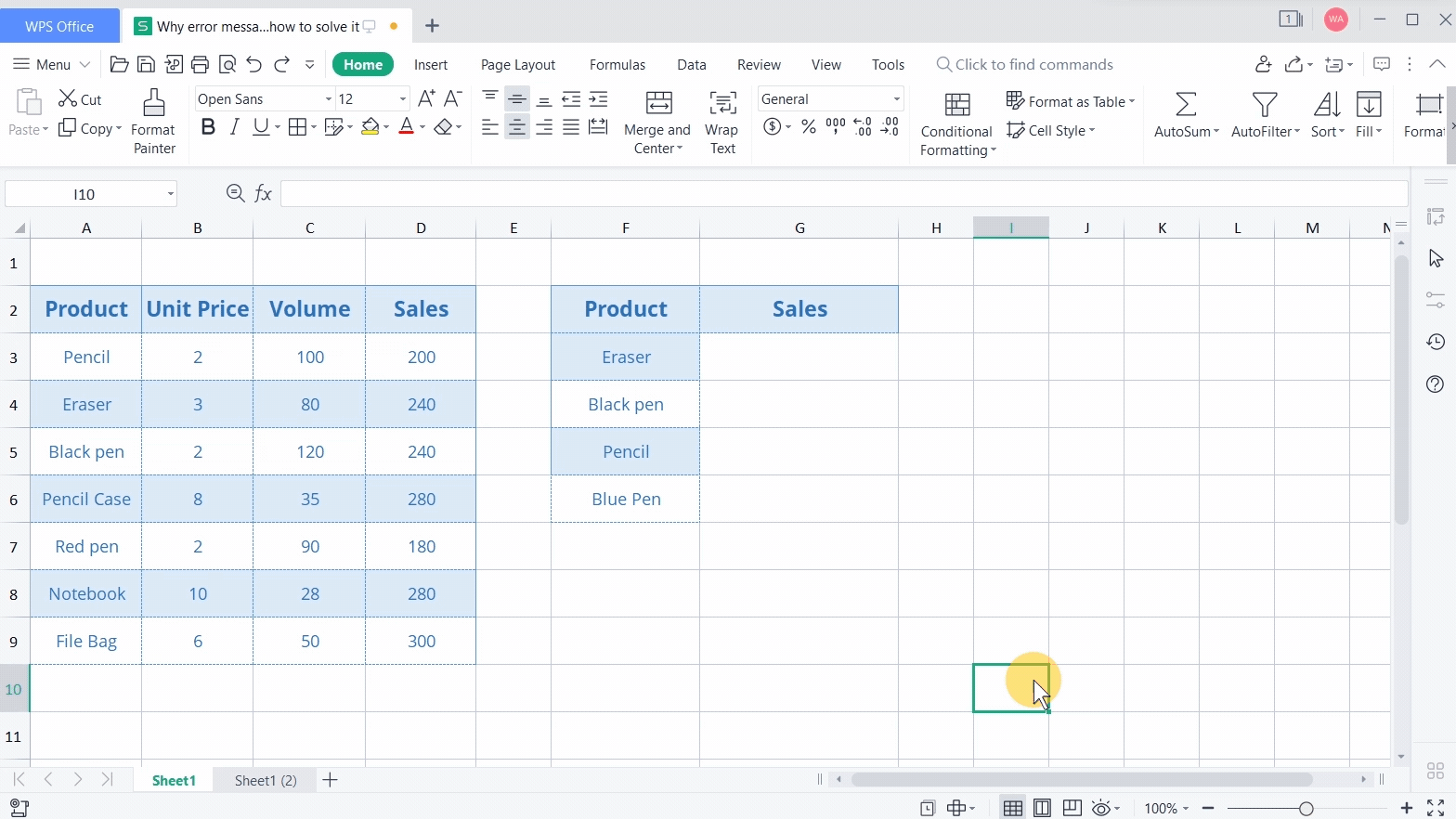The first reason: the searched value is not in the searching range.

Take this table as an example. To get the sales of erasers, black pens, pencils, and blue pens, we need to insert the following formula: =VLOOKUP(F3,A2:D9,4,0)

F3 is Lookup_value, Eraser is the value to look for. A2:D9 is Table_array, the entire table is the range containing the data to look for. 4 is Col_index_num, the column in the table from which the matching value must be returned, we enter 4 here because Sales locates in the fourth column. 0 means this formula will perform an exact matchFilling down these cells,  we will find #N/A in cells G5 and G6. This is because there is no lookup value in the specific range.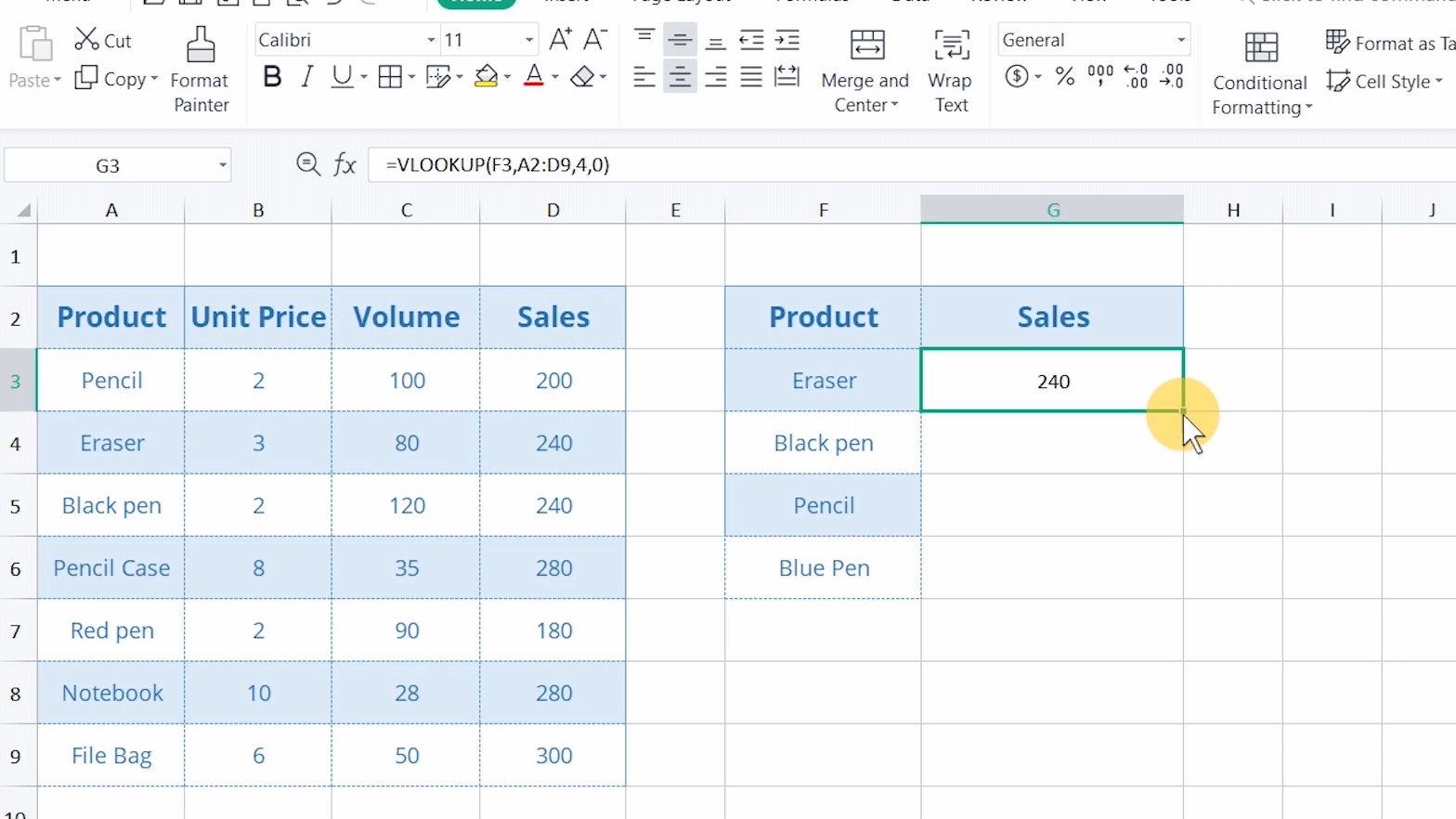After filling the formula, let's double-click cell G5. The data area A2:D9 will be copied as A4:D11, making Pencil find no results.

How can we fix it?

To solve this, we need to make the data range an absolute reference. Select the table array A2:D9, and press the shortcuts F4 to fix the range as an absolute reference.

When filling the formula again, we will get the pencil's sales.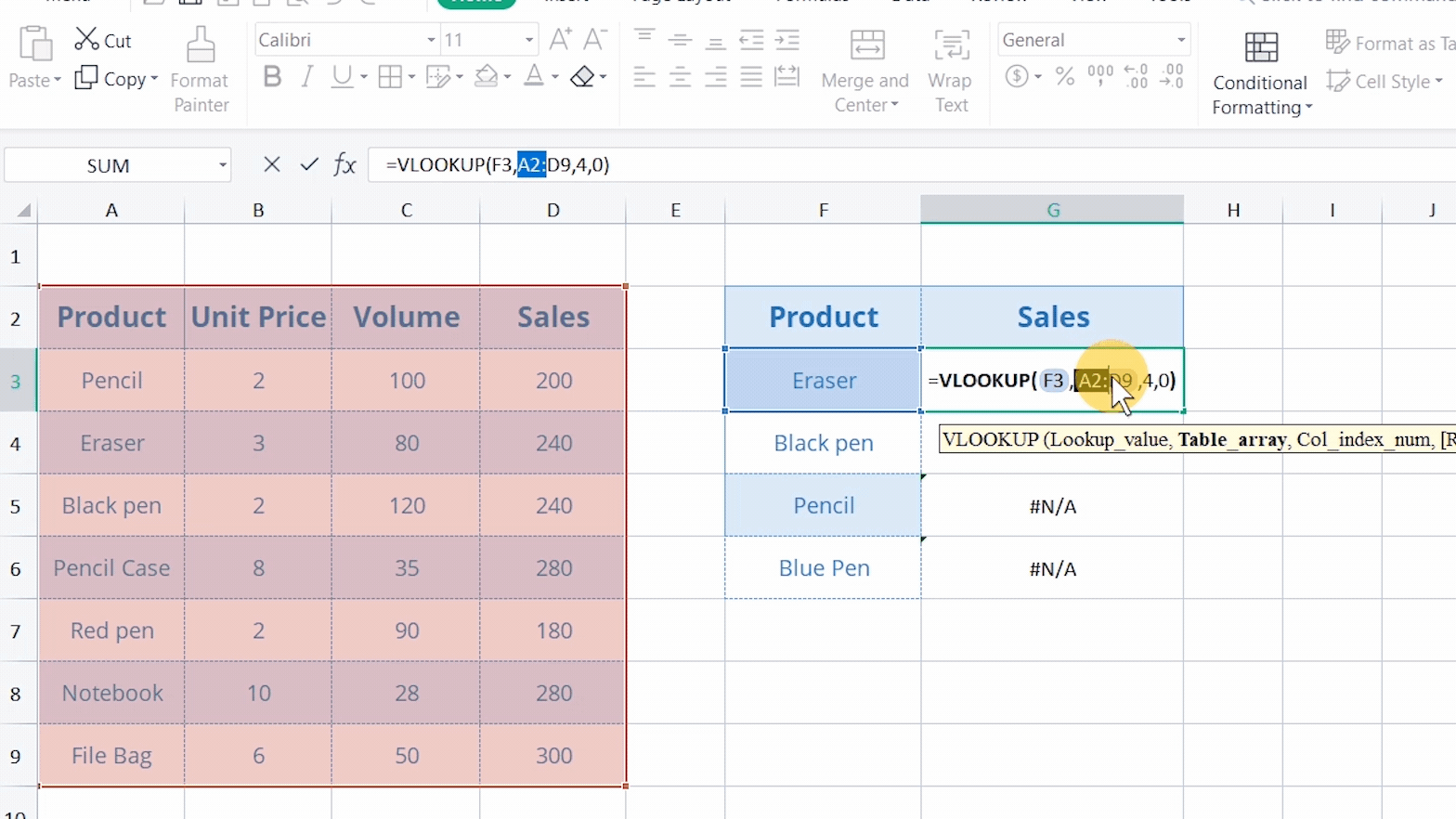However, the sales of the blue pen have yet to be found. This is because there is no data for the blue pen in the data source.

The second reason: a function with a return value of #N/A is referenced.

Suppose we want to get the total sales of all items here. Entering =SUM(G3:G6) will only bring us the #N/A result.

This is because in the cell G6 we put the formula that returns #N/A and refer it when calculating. To fix it, we can choose the IFERROR function so that the error value will be replaced by texts or numerical values.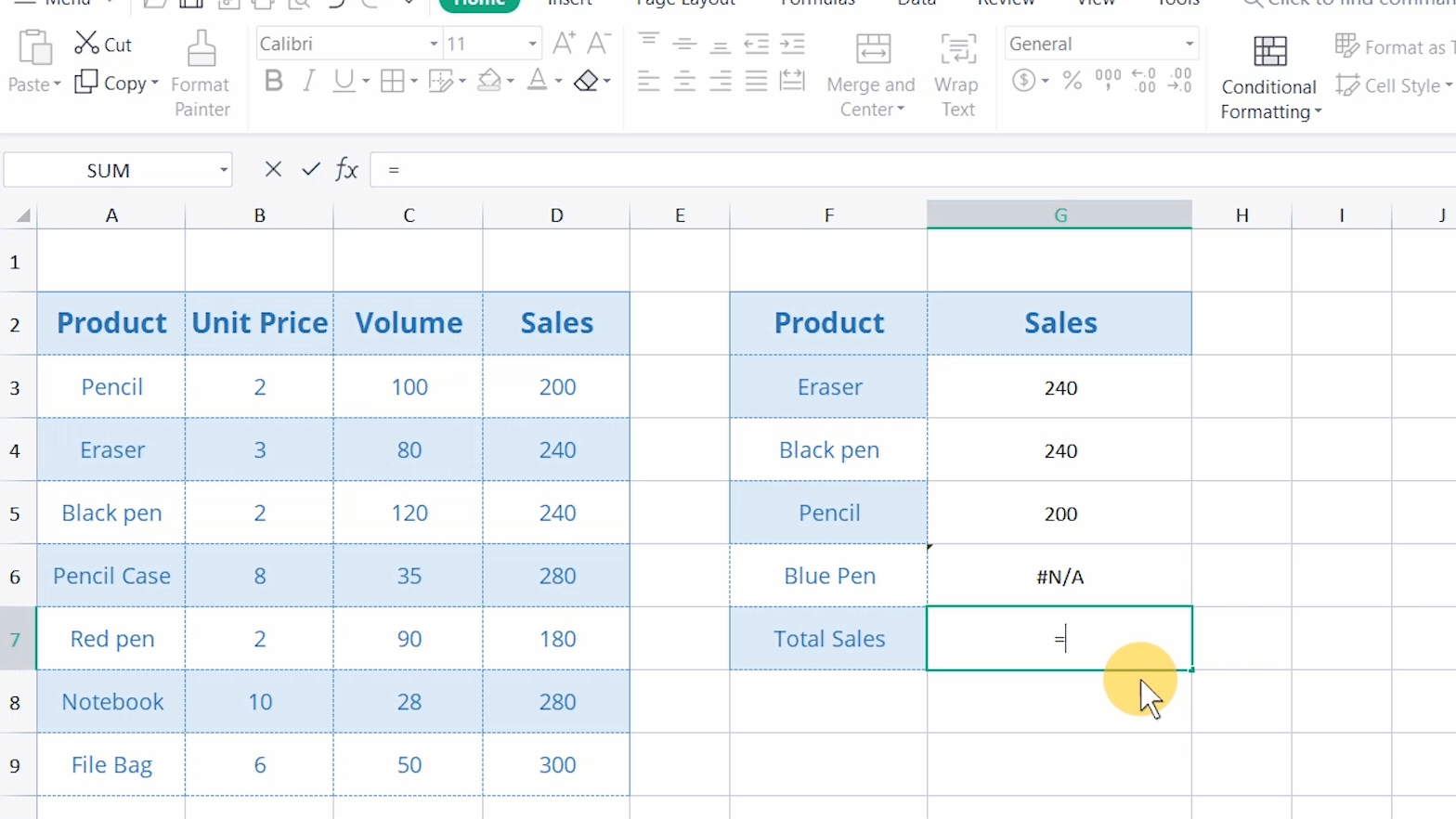Here, let's re-enter the formula as =IFERROR(VLOOKUP(F6,\$A\$2:\$D\$9,4,0),0). By doing so, when the VLOOKUP formula returns an error value, we will get a 0 as the final result. So no error will appear.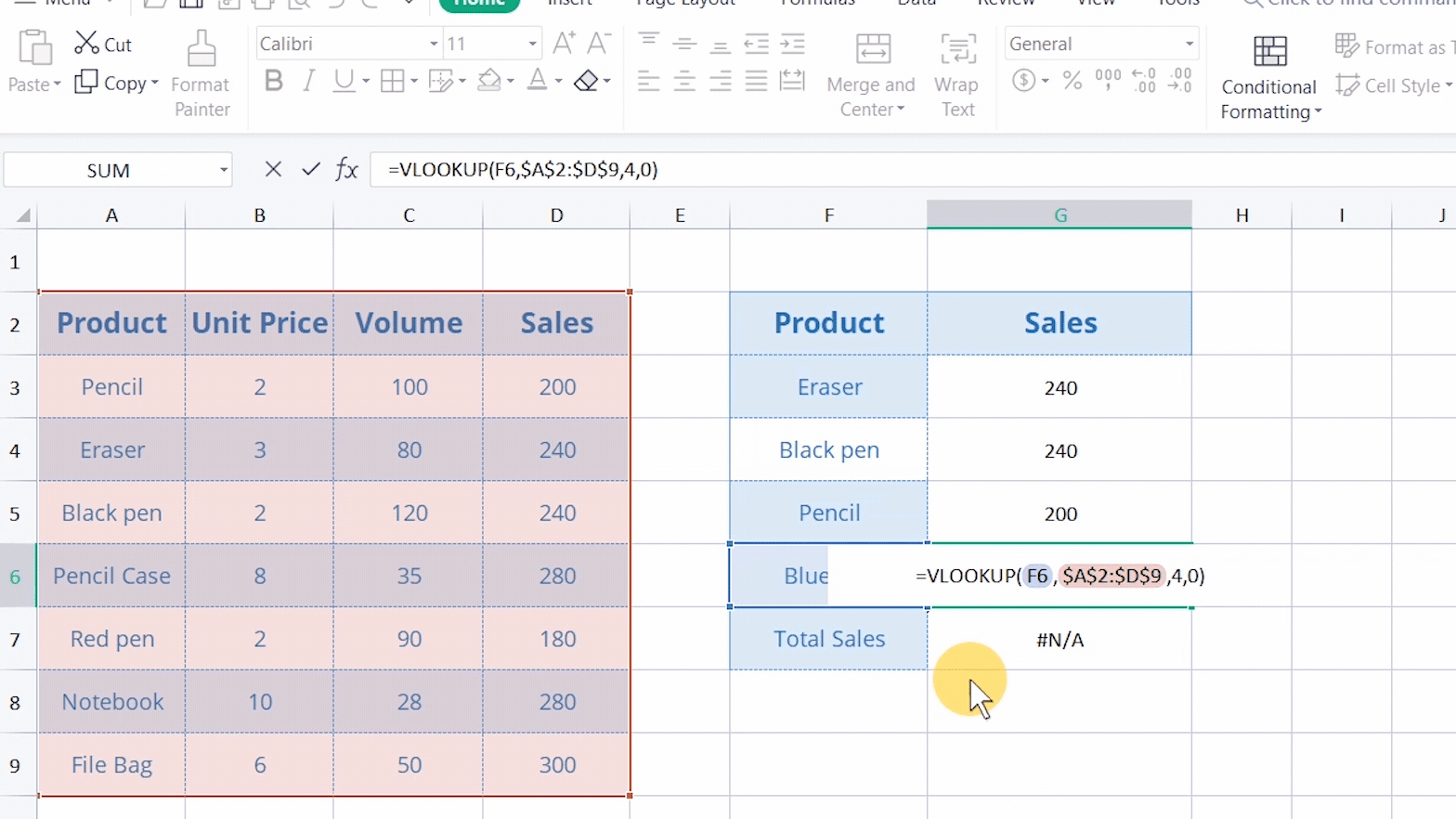WPS Spreadsheet is a powerful chart and form processor. In WPS Academy, you can learn not only how to use Spreadsheet to sort out data but also how to corporate numeric information into graphs. By watching the step-by-step tutorials on WPS Academy, you will become a spreadsheet expert soon.